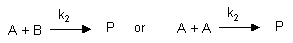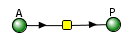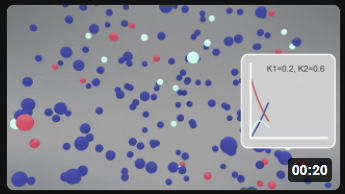# 6.2: Kinetics without Enzymes

$$\newcommand{\vecs}{\overset { \rightharpoonup} {\mathbf{#1}} }$$ $$\newcommand{\vecd}{\overset{-\!-\!\rightharpoonup}{\vphantom{a}\smash {#1}}}$$$$\newcommand{\id}{\mathrm{id}}$$ $$\newcommand{\Span}{\mathrm{span}}$$ $$\newcommand{\kernel}{\mathrm{null}\,}$$ $$\newcommand{\range}{\mathrm{range}\,}$$ $$\newcommand{\RealPart}{\mathrm{Re}}$$ $$\newcommand{\ImaginaryPart}{\mathrm{Im}}$$ $$\newcommand{\Argument}{\mathrm{Arg}}$$ $$\newcommand{\norm}{\| #1 \|}$$ $$\newcommand{\inner}{\langle #1, #2 \rangle}$$ $$\newcommand{\Span}{\mathrm{span}}$$ $$\newcommand{\id}{\mathrm{id}}$$ $$\newcommand{\Span}{\mathrm{span}}$$ $$\newcommand{\kernel}{\mathrm{null}\,}$$ $$\newcommand{\range}{\mathrm{range}\,}$$ $$\newcommand{\RealPart}{\mathrm{Re}}$$ $$\newcommand{\ImaginaryPart}{\mathrm{Im}}$$ $$\newcommand{\Argument}{\mathrm{Arg}}$$ $$\newcommand{\norm}{\| #1 \|}$$ $$\newcommand{\inner}{\langle #1, #2 \rangle}$$ $$\newcommand{\Span}{\mathrm{span}}$$

## Single Step Reactions

We will first explore the kinetics of non-catalyzed reactions, which is needed to understand the kinetic of the more complicated enzyme-catalyzed reaction. First a brief note.

##### Calculus: Derivatives and Integrals

In this book we will refer to the change of concentration of a chemical species X as a function of time as dX/dt instead of ΔX/Δt, where dX/dt is the derivative of X with respect to time t. This is the language of calculus, which most readers would have studied. The use of calculus will be mostly limited to writing equations of the form dX/dt = f(t), which is called differential equations. We will also use a few integrals, but a working knowledge of calculus is not required. For readers who have not studied calculus, just replace dX/dt with ΔX/Δt in your mind and you will derive the same meaning.

You studied two major types of kinetic equations in introductory chemistry to analyze kinetic data:

• initial velocity method: In this method, the initial rate, v0, is measured as a function of the concentration of reactants. The initial velocity, v0, is the initial slope of a graph of the concentration of reactants or products as a function of time, taken over a range of times such that only a small fraction of A has reacted. Under these condition, [A] over this short range of time is approximately constant and equal to Ao. Initial rate graphs are often based on measurement of product increase with time, ΔP/Δt, so v0 vs A plots have positive slopes. The velocity at time t along the A vs t curve, dA/dt, is constantly changing as [A] decreases since the velocity depend on the [A]. To reiterate, the initial velocity of the reaction is the slope of the initial linear part of the decay curve when the rate is essentially linear over a narrow range of A concentrations.
• integrated rate method: In this method a differential equation which gives the change of A or P with time (dA/dt or dP/dt) is integrated to give a rate equation giving the concentration of A or P as a function of time.

Most biochemistry textbooks focus on initial velocities when explaining enzyme kinetics. However, in many ways, this method is less intuitive than exploring how the concentration of a molecule changes with time. An analogy is an intuitive understanding of density (mass/volume), which is more complicated than understanding either mass or volume separately. We choose to use both methods to develop both chemical and biological intuitions of kinetic properties.

We will first explore simple irreversible reactions (1st and 2nd order), then make them reversible, and then couple them together to form more complex reaction schemes, much as we are compelled to do for enzyme-catalyzed reactions.

#### First Order Reaction:

$\ce{A ->[k_{1}] P} \nonumber$

where k1 is the first order rate constant. For these reactions, the velocity of the reaction, $$v$$, is directly proportional to [A], or

$v=-\frac{d A}{d t}=+\frac{d P}{d t}=k_{1} A \label{6.3.A.1}$

The negative sign in -d[A]/dt indicates that the concentration of A decreases. The equation could also be written as:$$v=\frac{d A}{d t}=-k_{1} A$$

For the rest of the reactions shown in this chapter, I will follow the convention of writing all velocities expressed as d[x]/dt as positive numbers. A negative sign for a term on the right hand side of the differential equation will indicate that the concentration dependency of that term will lead to a decrease in [x] with time. Likewise a positive sign for the term on the right hand side of the equation will indicate that concentration dependency of that term will lead to an increase in [x] with time.

The differential Equation 6.3.A.1 can solved to find [A] as a function of t.

\begin{aligned} \int_{A_{O}}^{A} \frac{d A}{A}=-k_{1} \int_{0}^{t} d t & \\ \ln A-\ln A_{0}=&-k_{1} t \\ \ln A=\ln A_{0}-k_{1} t \end{aligned} \nonumber

$A=A_{0} e^{-k_{1} t} \nonumber$

Equation 6.3.A.2 is an example of an integrated rate equation. The following graphs show plots of A vs t and lnA vs t for data from a first order process. Note that the derivative of the graph of A vs t (dA/dt) is the velocity of the reaction. The graph of lnA vs t is linear with a slope of -k1. The velocity of the reaction (slope of the A vs t curve) decreases with decreasing A, which is consistent with equation 1. Again, the initial velocity is determined from data taken in the first part of the decay curve when the rate is linear and little A has reacted. That is [A] is approximately equal to [A0].

Figure $$\PageIndex{1}$$ below shows two ways to plot 1st order reaction data. The left graph shows the exponential decay of A with time and the corresponding rise in P when A0 is 0 and k1=2. The other shows the linear fall of ln[A] vs time.Figure $$\PageIndex{1}$$: Two ways to plot 1st order reaction data
 Once again, for complete clarification, another way of analyzing the kinetics of a reaction, in addition to following the concentration of a reactant or product as a function of time and fitting the data to an integrated rate equation, is to plot the initial velocity, vo, of the reaction as a function of concentration of reactants. The initial velocity is the initial slope of a graph of the concentration of reactants or products as a function of time, taken over a range of times such that only a small fraction of A has reacted, so [A] is approximately constant = Ao. From the first order graph of A vs t above, the slope approaches 0 with increasing time as [A] approaches 0, which clearly indicates that the reaction velocity depends on A. For a first order process, two equivalent equations can be written showing the disappearance of A as v = -d[A]/dt = k1[A], while appearance of A as v = d[A]/dt = -k1[A], Both equations shows that v is directly proportional to A. As [A] is doubled, the initial velocity is doubled. Velocity graphs used by biochemists often show the initial velocity of product formation (not reactant decrease) as a function of reactant concentration. Hence, as the concentration of product is increasing, the slopes of initial velocity are positive. A graph of v (= dP/dt) vs [A] for a first order process would have a positive slope and be interpreted as showing that the rate of appearance of P depends linearly on [A].

#### Second Order/Pseudo First Order Reactionswhere $$k_2$$ is the second order rate constant. For the first of these irreversible reactions, the velocity of the reaction, v, is directly proportional to [A] and [B], or

$v= \dfrac{dA}{dt} = -k_2[A][B] \label{eq3}$

We will consider two special cases of this reaction type:

1. [B] >> [A]. Under these conditions, [B] never changes, so Equation \ref{3} becomes

4. v = -(k2[B]) [A] = -k1' [A]

where k1' is the pseudo first order rate constant (= k2[B] ) for the reaction. The reaction appears to be first order, depending only on [A].

1. The only reactant is A which must collide with another A to form P, as illustrated in the second reaction above. The derivations below apply to this special case.

The following differential equation can be written and solved to find [A] as a function of t.

$v=\frac{d A}{d t}=2 \frac{d P}{d t}=-k_{2} A^{2} \nonumber$

Solving the differential equation for A give the following:

$\begin{array}{c}{\frac{d A}{d t}=-k_{2} A^{2}} \\ {\int_{A_{0}}^{A} \frac{d A}{A^{2}}=\int_{A_{0}}^{A} A^{-2} d A=\int_{A_{0}}^{A} A^{-2} d A=-k_{2} \int_{0}^{t} d t} \\ {\left.\left.\left.\frac{A^{n+1}}{n+1}\right]_{A_{0}}^{A}=\frac{A^{-1}}{-1}\right]_{A_{0}}^{A}=-k_{2} t\right]_{A_{0}}^{A}} \\ {-\frac{1}{A}-\left(\frac{1}{A_{0}}\right)=-k_{2} t}\end{array} \nonumber$ or

$\frac{1}{A}=\frac{1}{A_{0}}+k_{2} t \nonumber$

Figure $$\PageIndex{2}$$ below show plots of A vs t and 1/A vs t for a second order process when A0 is 0 and k2=1. The right graph shows the linear rise of 1/[A] with time.Figure $$\PageIndex{2}$$: Plots of A vs t and 1/A vs t for a second order process when A0 is 0 and k2=1

Note that just from a plot of A vs t, it would be difficult to distinguish a first from second order reaction. If the plots were superimposed, you would observe that at the same concentration of A (10 for example), the vo of a first order reaction would be proportional to 10 but for a second order reaction to 102 or 100. Therefore, the second order reaction is faster (assuming similarity in the relative magnitude of the rate constants) as indicated by the steeper negative slope of the curve. However, at low A (0.1 example), the vo of a first order reaction would be proportional to 0.1 but for a second order reaction to 0.12 or 0.01. Therefore, at low A, the second order reaction is slower.

The interactive graphs below show the first and second order conversion of reactant A to product. Change the sliders to see how the curves are different.

By comparing these curves, you should be able to see how difficult it is to differentiate between a 1st and 2nd order process unless the reaction is allowed to progress to almost completion.

## Multi-Step Reactions

#### Reversible First Order ReactionsThe following differential equation for this reaction scheme can be written and solved as shown below:

\begin{aligned} \mathrm{v}=& \frac{\mathrm{d} \mathrm{A}}{\mathrm{dt}}=-\mathrm{k}_{\mathrm{f}} \mathrm{A}+\mathrm{k}_{\mathrm{r}} \mathrm{P} \\ & \mathrm{A}=\frac{\mathrm{A}_{0}\left(\mathrm{k}_{\mathrm{r}}+\mathrm{k}_{\mathrm{f}}\left[\mathrm{e}^{-\left(\mathrm{k}_{\mathrm{f}}+\mathrm{k}_{\mathrm{r}}\right) \mathrm{t}}\right]\right.}{\mathrm{k}_{\mathrm{f}}+\mathrm{k}_{\mathrm{r}}} \\ \mathrm{P}=& \mathrm{A}_{0}-\left(\frac{\mathrm{A}_{0}\left(\mathrm{k}_{\mathrm{r}}+\mathrm{k}_{\mathrm{f}}\left[\mathrm{e}^{-\left(\mathrm{k}_{\mathrm{f}}+\mathrm{k}_{\mathrm{r}}\right) \mathrm{t}}\right]\right.}{\mathrm{k}_{\mathrm{f}}+\mathrm{k}_{\mathrm{r}}}\right) \end{aligned} \nonumber

Figure $$\PageIndex{3}$$ below shows graphs of A and P vs t for this reaction at two different sets of values of k1 and k2.Figure $$\PageIndex{3}$$: Graphs of A and P vs t for the reversible reaction A↔P at two different sets of values of k1 and k2

Change the sliders on the interactive graph below of a reversibel reaction to see how changing the relative values of the forward and reverse rate constants affects the concentrations at which the concentration plateaus are reached.We all grew up on mathematical graphs that give you valuable insight into textual descriptions and data tables from which the graphs were made. These graphs are enhanced when you can use sliders to change constants as in the case for the reversible reaction A ↔ P above. Even then you might not infer that when the reaction has reached equilibrium, product is still being made from reactant and reactant from product since the equilibrium is dynamic. To add insight into both simple and complex reactions, animations that show the continual disappearance of reactants and products are valuable.

This book will incorporate many animation to show the continue dynamic reactions. We will use reactants/products concentrations curves as a function of time, often called progress curves, to create the animation. All of the animations in this book were made by Hui Liu and Shraddha Nayak (Animation Lab, University of Utah). Multiple modes of presentation are useful as learners with different backgrounds and preferred ways of learning attempt to understand complex materials.

It is easy to write the equations (differential) to show how the rate of disappearance of a reactant A (for example), dA/dt, depends on concentration of the immediate participants in the reaction. It is not so easy to solve the equations (as we did above) for the progress curve which shows how [A] changes with time t (i.e. [A] = f(t)). Luckily, many programs have been developed that produce numerical solutions to the differential equations and give progress curve graphs like [A] = f(t). Two interrelated, freely available programs include Copasi and Vcell, can be used to solve all the equations for hundreds of cellular reactions simultaneously. They use a format called Systems Biology Markup Language (SBML) for describing and storing computational models. We will use Vcell in this book as it is very simple to use. All of the coding to describe the reactions is built into this book and hidden from you. All you will see are the output results, but you will also be given the opportunity to change constants (much as you do with the sliders on the graphs directly incorporated into this book) and see the resulting changes in graphical outputs.

The VCell insert box below shows the reaction diagram (left) and graphical results of the progress curve (right) for the reversible reaction of A ↔ P for the conditions shown using VCell.

• Species: The reactant A and product P are called species and are shown as green spheres.
• Reaction Node: The yellow square is a reaction node indicating a reaction connects A to P.
• Lines: The lines connect the species that participate in the reaction. The arrows appear unidirectional BUT the equations describing the concentrations of A and P are derived assuming a reversible reaction with rate constants kf (forward reaction) and kr (reverse reaction).
• Fluxes: The velocities (slope of the concentration vs time curve at any given time) are called fluxes, J, in Vcell and many other similar programs. When we get to metabolism, we will talk about fluxes of metabolites through pathways. Also fluxes are used to describe the rate of movement of solute through membranes.
• Progress Curves: These show A and P (in different colors) vs time.
#####Reversible Rx A ↔ B: kF=2, kR=4

Vcell OMEX-SBML file

Model:Parameters: kf = 0.2, kr = 0.4, A t=0 = A0 = 10

Animations

The video animations shows particles representing A (red) and P (cyan) interconverting in a reversible process with imbedded progress curves showing A (red) and P (cyan) vs time.

 Reversible Rx A (red) ↔ B (cyan): kF = kR = 3 Reversible Rx A (red) ↔ B (cyan): kF=4, kR=2 Reversible Rx A (red) ↔ B (cyan): kF=2, kR=4#### Consecutive First Order ReactionsThe following differential equations can be written for these reactions:

\begin{aligned} \frac{\mathrm{d} \mathrm{A}}{\mathrm{dt}} &=-\mathrm{k}_{1} \mathrm{A} \\ \frac{\mathrm{d} \mathrm{B}}{\mathrm{dt}}=& \mathrm{k}_{1} \mathrm{A}-\mathrm{k}_{2} \mathrm{B} \\ \frac{\mathrm{d} \mathrm{C}}{\mathrm{dt}} &=\mathrm{k}_{2} \mathrm{B} \end{aligned} \nonumber

Here are the solutions to the differential equations:

\begin{array}{c}{\mathrm{A}=\mathrm{A}_{0} \mathrm{e}^{-\mathrm{k}_{1} \mathrm{t}}} \\ {\mathrm{B}=\frac{\mathrm{k}_{1} \mathrm{A}_{0}}{\mathrm{k}_{2}-\mathrm{k}_{1}}\left(\mathrm{e}^{-\mathrm{k}_{1} \mathrm{t}}-\mathrm{e}^{-\mathrm{k}_{2} \mathrm{t}}\right)} \\ {\mathrm{C}=\mathrm{A}_{0}-\mathrm{A}-\mathrm{B}=\mathrm{A}_{0}\left[1+\frac{1}{\mathrm{k}_{1}-\mathrm{k}_{2}}\left(\mathrm{k}_{2} \mathrm{e}^{-\mathrm{k}_{1} \mathrm{t}}-\mathrm{k}_{1} \mathrm{e}^{-\mathrm{k}_{2} \mathrm{t}}\right]\right.}\end{array}

Figure $$\PageIndex{4}$$ below shows graphs of A, B, and C vs t for these reaction for a fixed value of k1 and k2.Figure $$\PageIndex{4}$$: Graphs of A, B, and C vs t for the irreversible reactions A → B → C for a fixed value of k1 and k2.

Change the sliders on the interactive graph below to see how changing the relative values of the forward and reverse rate constants affects the the curves.

You can imagine that deriving the equations for the completely reversible reactions of A ↔ B ↔ C would be really difficult. No problem! We can simply set up the reactions in Vcell, choice the build in equation based on mass action, and let the program numerically solve the equations to give progress curve graphs.

Let's run two different Vcell simulations, one for the irreversible reactions of A → B → C and one for the reversible reactions A ↔ B ↔ C.

##### Arrows in Vcell wiring diagram

In the reaction diagram below, the arrows goes in only one direction, left to right, simply showing that species are connected. In the Vcell program, the equations for the reversible reaction were used for both cases, but for the irreversible reaction, the rate constants for the backwards reaction were set to 0.

#####Irreversible reaction A → B → C. Default parameters: k1 = 0.2, k2 = 0.6, A t=0 = A0 = 1

Vcell OMEX-SBML file

Model:Here are two different animations for the irreversible reaction made using different rate constants.

 Irreversible Rx A (red) → B (cyan) → C (blue) k1 = 0.2, k2 = 0.6 Irreversible Rx A (red) → B (cyan)→ C (blue) k1= 0.6, k2 = 0.2Now let's look at the simulation for the fully reversible reactions A ↔ B ↔ C for which solving the differential equation would be much harder. Let VCell use numerical methods to make it easy!Reversible reaction A ↔ B ↔ C. Default parameters: k1f = 0.2, k1r = 0.1, k2f = 0.6, k2r = 0.3 A0 = 1

Vcell OMEX-SBML

Model:Here is the corresponding animation for the fully reversible reaction A ↔ B ↔ C.

 Reversible Rx A (red) ↔ B (cyan) ↔ C Blue) k1f = 0.2, k1r = 0.1; k2f =0.6, k2r = 0.36.2: Kinetics without Enzymes is shared under a not declared license and was authored, remixed, and/or curated by Henry Jakubowski and Patricia Flatt.